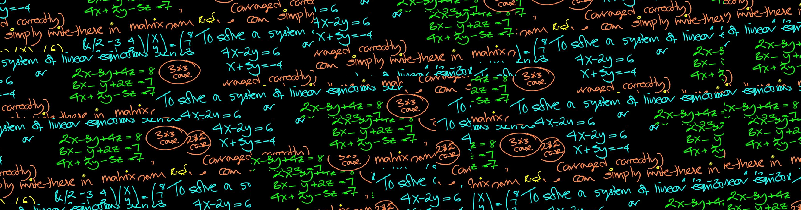# Gaussian elimination method – case of indefinite systemIn previous sections you can find theoretical background and examples including application of matrix representation and example of linear system having no solutions. As we already said, if you’re solving a system of linear equations there are three possible situations: there’s either unique solution, or infinitely many solutions, or no solution at all. Obviously, in your homework you potentially can obtain any of those when solving systems of linear equations, so that’s a nice idea to know how to deal with each of them. In this section we’re going to discuss the case when system of linear equations has infinitely many solutions.

Let’s solve the following system of linear algebraic equations:

\left\{ \begin{aligned}2x_1-x_2+3x_3=4\\-3x_1+2x_2+x_3=5\\-4x_1+2x_2-6x_3=-8 \end{aligned}\right.

As you could notice, this system differs from the previous example only in the right part of the third equation (we have $-8$ instead of $1$). Yet this will lead to a totally different result.

Here’s video version of this tutorial:

For convenience we consider matrix of coefficients for our system:

$$A=\begin {pmatrix} 2&-1&3 \\-3& 2&1 \\-4& 2&-6 \end {pmatrix}, \vec{b}=\begin{pmatrix}4\\5\\-8\end{pmatrix},\vec{x}=\begin{pmatrix}x_1\\x_2\\x_3\end{pmatrix}, \tilde{A}=\begin{pmatrix}2&-1&3&|&4 \\-3& 2&1&|&5 \\-4& 2&-6 &|&-8 \end{pmatrix}$$

Thus, the given system is represented as follows:

$$A \vec{x}=\vec{b}$$

As we’re going to apply Gaussian elimination method, our goal is to transform initial system so it takes the triangular (or echelon) form. Our possible actions are to swap rows of matrix, add or subtract them, multiply or divide by real non-zero number. Again, as we have non-zero right part of the system, i.e. our system is not homogeneous, augmented matrix $\tilde{A}$ appears in the game:

$$\tilde{A}=\begin{pmatrix}2&-1&3&|&4 \\-3& 2&1&|&5 \\-4& 2&-6 &|&-8 \end{pmatrix}$$

Divide the first row by $2$ in order to obtain coefficient $1$ in front of $x_1$:

$$\begin{pmatrix}1&-\frac{1}{2}&\frac{3}{2}&|&2 \\-3& 2&1&|&5 \\-4& 2&-6 &|&-8 \end{pmatrix}$$

Next is to eliminate the first unknown $x_1$ from the second and the third equations. For that, subtract the first row multiplied by $(-3)$ from the second:

$$\begin{pmatrix}1&-\frac{1}{2}&\frac{3}{2}&|&2 \\0& \frac{1}{2}&\frac{11}{2}&|&11 \\-4& 2&-6 &|&-8 \end{pmatrix}$$

Then we multiply the second row by $2$ (doing so we obtain coefficient $1$ in front of $x_2$):

$$\begin{pmatrix}1&-\frac{1}{2}&\frac{3}{2}&|&2 \\0& 1&11&|&22 \\-4& 2&-6 &|&-8 \end{pmatrix}$$

Ok, now we move on to the third row. To eliminate $x_1$ from it we subtract the first row times $(-4)$ from the  third row:

$$\begin{pmatrix}1&-\frac{1}{2}&\frac{3}{2}&|&2 \\0& 1&11&|&22 \\0&0&0 &|&0 \end{pmatrix}$$

Look what we’ve obtained in the third row of our matrix (which stands for the third equation): all coefficients are zeros! Actually, this is equivalent to the equation $0=0$. This is correct, yet provides no information about unknowns, so we just drop this equation out of the consideration. Thus, our system degenerates into one with only two equations:

$$\begin{pmatrix}1&-\frac{1}{2}&\frac{3}{2}&|&2 \\0& 1&11&|&22 \end{pmatrix}$$

Now we have system of two equations with three unknowns. Such systems are called indefinite because we can’t find exact values of the unknowns, we only can express two of them through the third which is called free variable. Let’s say, for example, that $x_3$ is free variable. Then we express $x_1, x_2$ through $x_3$.

Let’s subtract the second row divided by $(-2)$ from the first row:

$$\begin{pmatrix}1&0&7&|&13 \\0& 1&11&|&22 \end{pmatrix}$$

Returning to equations, we have:

\left\{ \begin{aligned}x_1+7x_3=13\\x_2+11x_3=22 \end{aligned}\right.

whence

\left\{ \begin{aligned}x_1=-7x_3+13\\x_2=-11x_3+22 \end{aligned}\right.

and that’s the answer. We cannot uniquely define the values of unknowns in this case. Such systems of equations are called indefinite. Value of the third unknown $x_3$ is arbitrary real number, this means that there are infinitely many solutions of this system. Note that we could have chosen another variable to be free variable and express two others through it, that would also be correct.When you’re finished, don’t forget to check your results. For that you can substitute obtained values into the initial system. If you obtain right equalities instead of equations then your solution is correct. Also you can use online system solver. Do not overuse it, though, as on your finals you won’t be allowed to apply such stuff.

Have math questions, cannot deal with homework tasks on your own? Ask questions and get free homework answers. More math in our next sections!

0 Shares
Filed under Math.